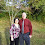## December 12, 2012

### Laws of Sines and Cosines

Honors PreCalculus is essentially a new prep for me (although there was that one year where I taught a combination PreCalc/Calc class...) and I'm not a big fan of our textbook, so this unit was the first one I was really happy with.  We started the year with trigonometry and went through radians, unit circles, graphing and identities.  By the time we finished identities students were desperate to solve an equation, any equation, and get a number as an answer.  Enter, law of sines and law of cosines.  I wanted students to prove the laws before they used them, so I searched through my pile of textbooks and found a nice sequence for law of sines in the Discovering Geometry book.  It offered more scaffolding than I wanted to give students, so I shortened the procedure and put the pieces I took out on hint cards.  For law of cosines I couldn't find an investigation I liked in any of the books I have, so I turned to the internet.  Illuminations has a nice activity that I again modified to remove some of the scaffolding to hint cards, where only students who needed the extra help would get it.  I thought it was important for students to see the standard form of law of cosines because it makes the formula so much easier to memorize, and the beauty of its symmetry as well as its association with the pythagorean theorem jump out at you.  The hint cards worked great for students who were absent from class as well; when students work together they each figure out different parts of the problem, but without someone to bounce ideas off of they relied more on the hints.  Those students were able to work independently after school while I helped other students, which was a huge help on the super busy days.

law of sines, cosines

Once students had proven the laws, it was time to practice them.  One of the complaints I have about my textbook is the sheer lack of problems.  There aren't enough problems on any given topic to both practice in class and review for homework.  This unit I started projecting problems during class for students to solve.  I've been hesitant to do this in the past since students don't work at the same pace.  There are a million reasons why someone might work slower and I don't like rushing anyone through problems.  The students, however, loved this format.  They all want to know instantly whether they got the right answer, and before I changed each slide I would write up the correct answers for students to check their work.  They're also big fans of not carrying a huge textbook around.  I found that by the time some students had finished the four problems on a slide, everyone else had finished at least two.  And if I gave the students who finished first the responsibility of helping others they would be content waiting a couple extra minutes (and those minutes wouldn't be wasted since they were working with other students).  This format also keeps the momentum going so students see at least a couple of each type of problem.  I've mostly been using kuta worksheets, but have had to google for random worksheets when they don't cover a topic (which got me in trouble once since a test problem I pulled from a random site was impossible! Luckily I realized this before the test, but sadly after I'd copied it.)

Once students were comfortable solving for both sides and angles using both formulas, we looked into some applications.  I had students draw different triangles using rulers and protractors to introduce ambiguous triangles.  The book goes into 6 different cases (acute, obtuse, h<a, h>a etc.) which is far too many for my brain to consider at once.  Instead, my approach is: try to solve the triangle and see what happens!  If we get an equation with no solution, it's impossible.  If we get an equation with two solutions, try them both to see if each makes sense.  Voila, we have determined if there are 2 triangles, 1 triangle or no triangles.  Reasoning and sense making have much higher value in my classroom than memorization.  Though I did expect students to have memorized law of sines and law of cosines, they didn't complain about it at all because after doing a significant number of practice problems, everyone learned the equations by heart.

Finally, we looked at area of triangles.  I remembered to do $\frac{1}{2}ab\text{ sin}(C)$ but forgot Hero(n)'s formula until after the test.  We derived the first equation, but the second is just too tedious.  Instead I had students find the area given 3 sides (so they used the law of cosines followed by  $\frac{1}{2}ab\text{ sin}(C)$) once, asked them to evaluate $T=sqrt{s(s-xa)(s-b)(s-c)}$ and they said "aha! that's the area!"  I explained that they were perfectly capable of proving that formula as well, but it involved finding the difference of squares several times over and I figured I'd save them the effort.  Some students were interested in more detail, others responded "Thank goodness for Heron!"

I enjoyed this unit since it was a nice balance of abstract and concrete.  We drew actual triangles and talked about what made sense in the context of a triangle (I hope that these students still expect math to make sense), but also derived formulas.  To review for the test (and meet my quarterly writing prompt requirement) I had students describe what you could use the law of sines and the law of cosines for.  I have yet to read those or grade their tests (procrastination is an issue lately) but the quizzes went great.  Nearly every student earned a 3 or a 4, and the threes were typically because they didn't specify needing an opposite pair when describing "What information do you need to use the law of sines?"

1.2.